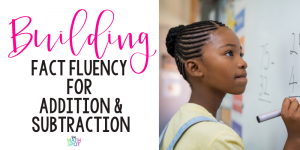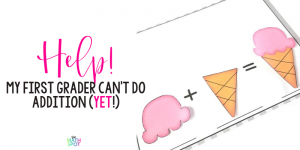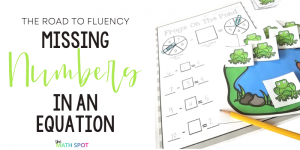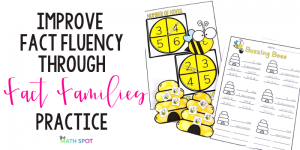# Category: Operations### The Simple & Effective Guide To Understanding Math Facts

Addition & Subtraction Strategies. Fact Fluency.   Fact Memorization.   Three different sides of the same coin. Thinking about teaching math facts as being learned at each of these three levels will change your perspective on instruction altogether! Addition & Subtraction Strategies Counting on fingers, using manipulatives, using a number

### How to Introduce Subtraction

There are a number of misconceptions and errors we can predict when we introduce subtraction to our students. Not understanding that subtraction starts with the total. Not understanding that subtraction can take away or take apart or find the difference. Not understanding that subtraction isn’t just the operation you useYou’ve spent the first week of the school year settling in with your students and you’re ready to dive into your math curriculum. The first unit is a quick review of “counting-all” addition, and this time next week you will be knee-deep in “counting on”. Somehow it’s the first day### Fluency Hack: Prioritizing Missing Numbers In Equations

If you are looking to increase your students’ fact fluency, prioritizing the skill of missing numbers in equations is one of the keys to unlocking that skill in your student. If your students have struggled with this skill in the past, you’re not alone! Solving for missing numbers in equations